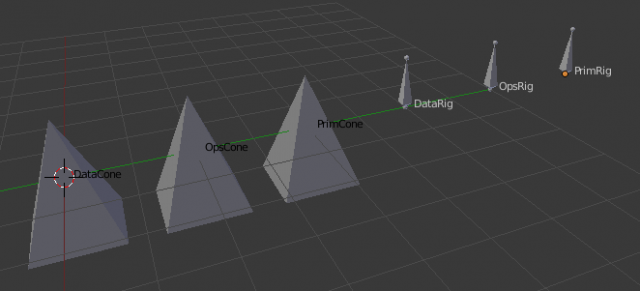# Three ways to create objects

The examples covered so far show that object can be created from Python using different paradigms.

## Data method

The data method closely mimics how data are stored internally in Blender.

• Add the data, and then the object. For a mesh:
```me = bpy.data.meshes.new(meshName)
ob = bpy.data.objects.new(obName, me)
```

and for an armature:

```amt = bpy.data.armatures.new(amtname)
ob = bpy.data.objects.new(obname, amt)
```
• Link the object to the current scene and make it active. Optionally, we can make the newly created object active or selected. This code is the same for all kinds of objects.
```scn = bpy.context.scene
scn.objects.active = ob
ob.select = True
```
• Fill in the data. In the mesh case, we add the lists of vertices and faces.
```me.from_pydata(verts, [], faces)
```

In the armature case, we switch to edit mode and add a bone.

```bpy.ops.object.mode_set(mode='EDIT')
bone = amt.edit_bones.new('Bone')
bone.tail = (0,0,1)
```
• Finally, it is usually necessary to update the modified data. In the mesh case, we call an update function explicity.
```me.update()
```

The armature is implicitly update when we switch to object mode.

```bpy.ops.object.mode_set(mode='OBJECT')
```

## Operator method

The operator method adds an object and a data block at the same time. The data block is currently empty, and needs to be filled with actual data later.

• Add the object with the `bpy.ops.object.add` operator. This automatically takes care of several things that we had to do manually in the data method: it creates object data (i.e. the mesh or armature), links the object to the scene, makes it active and selects the object. On the other hand, we must now retrieve the object and its data. This is straightforward because `bpy.context.data` always points to the active object.
To add a mesh object, we do
```bpy.ops.object.add(type='MESH')
ob = bpy.context.object
me = ob.data
```

```bpy.ops.object.add(
type='ARMATURE',
enter_editmode=True,
location=origin)
ob = bpy.context.object
amt = ob.data
```
• As in the data method, the actual data must be filled in and updated before use. For a mesh we add the verts and faces:
```me.from_pydata(verts, [], faces)
me.update()
```

and for an armature we add a bone:

```bone = amt.edit_bones.new('Bone')
bone.tail = (0,0,1)
bpy.ops.object.mode_set(mode='OBJECT')
```

Note that we do not need to explicitly enter edit mode, because the armature entered edit mode already on creation.

## Primitive method

If we want to make an object of a primitive type, there may exist an operator which creates the primitive with the desired properties.

• A cone is in fact approximated by a pyramid.

To create a pyramid mesh with 4 sides:

```bpy.ops.mesh.primitive_cone_add(
vertices=4,
depth=1,
cap_end=True)
```

whereas the following code adds a armature with a single bone;

```bpy.ops.object.armature_add()
bpy.ops.transform.translate(value=origin)
```
• As in the operator method, we then retrieve the newly create object from `bpy.context.object`.
```ob = bpy.context.object
me = ob.data
```

## Comparison

The primitive method is simplest, but it only works when a suitable primitive is available. Even in the example program, it creates a pyramid mesh which is slightly different from the other two methods; the base is not a single quad, but rather consists of four triangles with a common point in the middle of the base. The other two methods are more or less equivalent.

A primitive does not need to be particularly simple; there are primitives for creating a monkey mesh or a human rig. But the primitive method is always limited to prefabricated objects.

We use all three methods in the examples in this note.```#----------------------------------------------------------
# File objects.py
#----------------------------------------------------------
import bpy
import mathutils
from mathutils import Vector

def createMeshFromData(name, origin, verts, faces):
# Create mesh and object
me = bpy.data.meshes.new(name+'Mesh')
ob = bpy.data.objects.new(name, me)
ob.location = origin
ob.show_name = True

# Link object to scene and make active
scn = bpy.context.scene
scn.objects.active = ob
ob.select = True

# Create mesh from given verts, faces.
me.from_pydata(verts, [], faces)
# Update mesh with new data
me.update()
return ob

def createMeshFromOperator(name, origin, verts, faces):
type='MESH',
enter_editmode=False,
location=origin)
ob = bpy.context.object
ob.name = name
ob.show_name = True
me = ob.data
me.name = name+'Mesh'

# Create mesh from given verts, faces.
me.from_pydata(verts, [], faces)
# Update mesh with new data
me.update()
# Set object mode
bpy.ops.object.mode_set(mode='OBJECT')
return ob

def createMeshFromPrimitive(name, origin):
vertices=4,
depth=1,
cap_end=True,
view_align=False,
enter_editmode=False,
location=origin,
rotation=(0, 0, 0))

ob = bpy.context.object
ob.name = name
ob.show_name = True
me = ob.data
me.name = name+'Mesh'
return ob

def createArmatureFromData(name, origin):
# Create armature and object
amt = bpy.data.armatures.new(name+'Amt')
ob = bpy.data.objects.new(name, amt)
ob.location = origin
ob.show_name = True

# Link object to scene and make active
scn = bpy.context.scene
scn.objects.active = ob
ob.select = True

# Create single bone
bpy.ops.object.mode_set(mode='EDIT')
bone = amt.edit_bones.new('Bone')
bone.tail = (0,0,1)
bpy.ops.object.mode_set(mode='OBJECT')
return ob

def createArmatureFromOperator(name, origin):
type='ARMATURE',
enter_editmode=True,
location=origin)
ob = bpy.context.object
ob.name = name
ob.show_name = True
amt = ob.data
amt.name = name+'Amt'

# Create single bone
bone = amt.edit_bones.new('Bone')
bone.tail = (0,0,1)
bpy.ops.object.mode_set(mode='OBJECT')
return ob

def createArmatureFromPrimitive(name, origin):
bpy.ops.transform.translate(value=origin)
ob = bpy.context.object
ob.name = name
ob.show_name = True
amt = ob.data
amt.name = name+'Amt'
return ob

def run(origo):
origin = Vector(origo)
(x,y,z) = (0.707107, 0.258819, 0.965926)
verts = ((x,x,-1), (x,-x,-1), (-x,-x,-1), (-x,x,-1), (0,0,1))
faces = ((1,0,4), (4,2,1), (4,3,2), (4,0,3), (0,1,2,3))

cone1 = createMeshFromData('DataCone', origin, verts, faces)
cone2 = createMeshFromOperator('OpsCone', origin+Vector((0,2,0)), verts, faces)
cone3 = createMeshFromPrimitive('PrimCone', origin+Vector((0,4,0)))

rig1 = createArmatureFromData('DataRig', origin+Vector((0,6,0)))
rig2 = createArmatureFromOperator('OpsRig', origin+Vector((0,8,0)))
rig3 = createArmatureFromPrimitive('PrimRig', origin+Vector((0,10,0)))
return

if __name__ == "__main__":
run((0,0,0))
```